# sklearn.datasets.make_moons¶

sklearn.datasets.make_moons(n_samples=100, shuffle=True, noise=None, random_state=None)[source]

Make two interleaving half circles

A simple toy dataset to visualize clustering and classification algorithms. Read more in the User Guide.

Parameters: n_samples : int, optional (default=100) The total number of points generated. shuffle : bool, optional (default=True) Whether to shuffle the samples. noise : double or None (default=None) Standard deviation of Gaussian noise added to the data. random_state : int, RandomState instance or None (default) Determines random number generation for dataset shuffling and noise. Pass an int for reproducible output across multiple function calls. See Glossary. X : array of shape [n_samples, 2] The generated samples. y : array of shape [n_samples] The integer labels (0 or 1) for class membership of each sample.

## Examples using sklearn.datasets.make_moons¶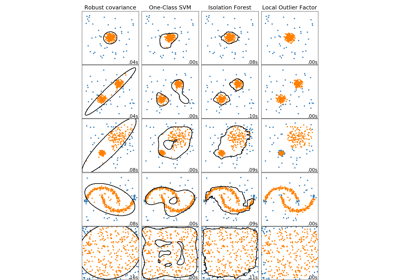Comparing anomaly detection algorithms for outlier detection on toy datasets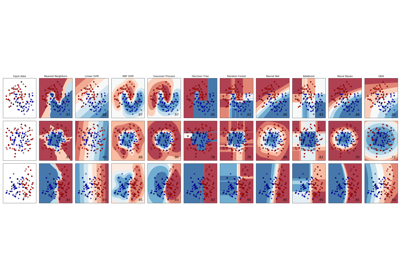Classifier comparison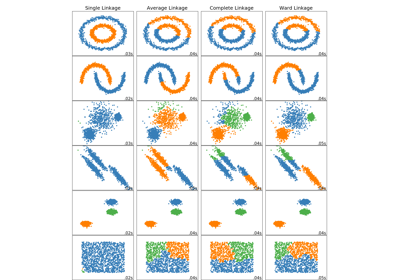Comparing different hierarchical linkage methods on toy datasets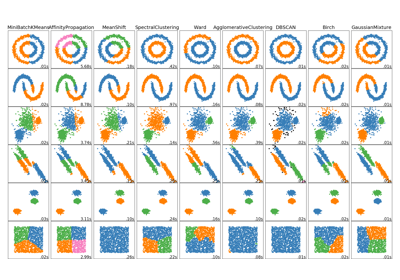Comparing different clustering algorithms on toy datasets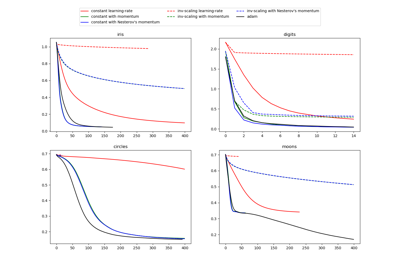Compare Stochastic learning strategies for MLPClassifier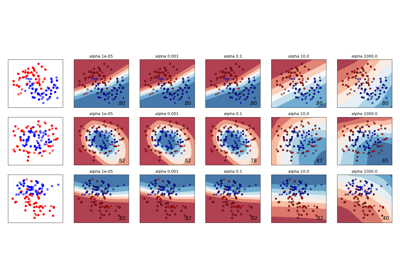Varying regularization in Multi-layer Perceptron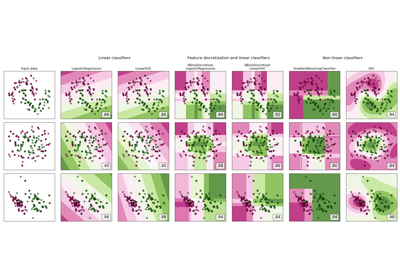Feature discretization